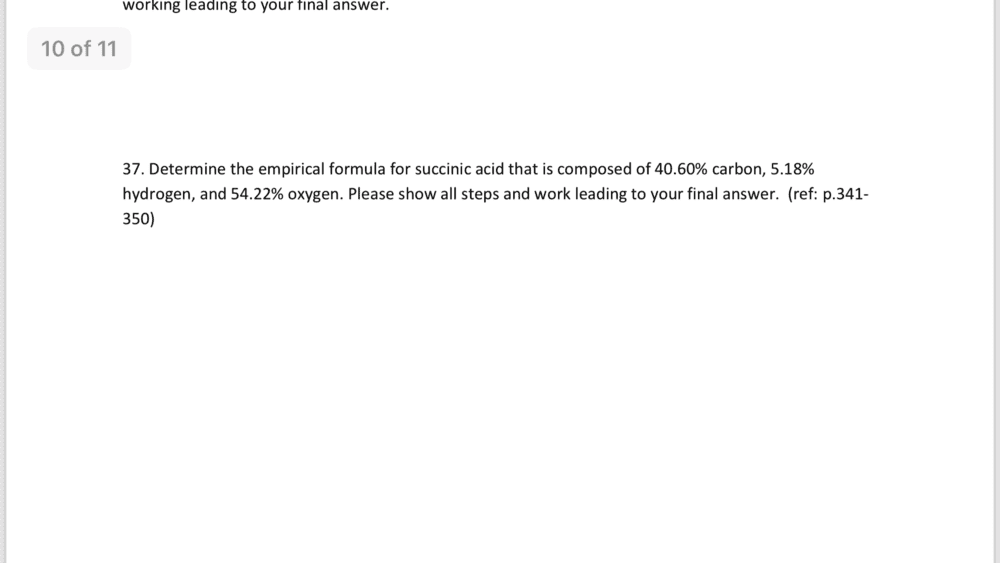# Empirical formula

Homework Statement:
I need help with a question
Relevant Equations:
N/A
Is everything correct?
Percent by mass C = 40.60%
Percent by mass H = 5.18%
Percent by mass O = 54.22%
Empirical formula = ? (unknown)

Inverse molar mass C = 1 mol/12.01 g
Inverse molar mass H = 1 mol/1.008 g
Inverse molar mass O = 1 mol/16.00 g

Convert the masses to moles.
C = 40.60 g/12.01 g/mol = 3.380 mol
H = 5.18 g/1.008 g/mol = 5.138 mol
O = 54.22 g/16.00 g/mol = 3.388 mol

Divide by the lowest, seeking the smallest whole-number ratio
C = 3.380/3.380 = 1 = 1 mol C
H = 5.138/3.380 = 1.52 = 1.5 mol H
O = 3.388/3.380 = 1.00 = 1 mol O
The simplest mole ratio is (1 mol C):(1.5 mol H):(1 mol O).

2 x 1 mol C = 2 mol C
2 x 1.5 mol H = 3 mol H
2 x 1 mol O = 2 mol O
The simplest whole-number ratio of atoms is (2 atoms C):(3 atoms H):(2 atoms O). The empirical formula of succinic acid is C2H3O2.## Answers and Replies

Homework Helper
Gold Member
That is a textbook answer to that problem!

That is a textbook answer to that problem!

Homework Helper
Gold Member
Like you authored the answer in the textbook’s solution manual!Like you authored the answer in the textbook’s solution manual!I followed an example in my book but I’m not sure if it’s correct..

•